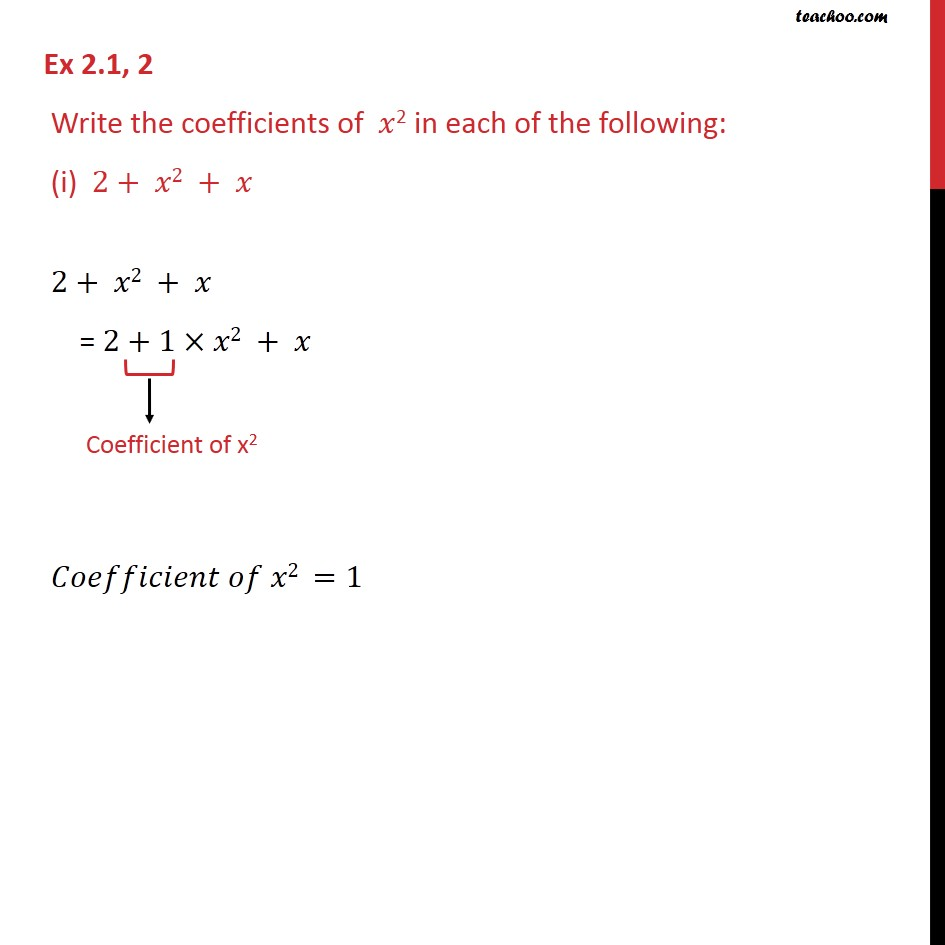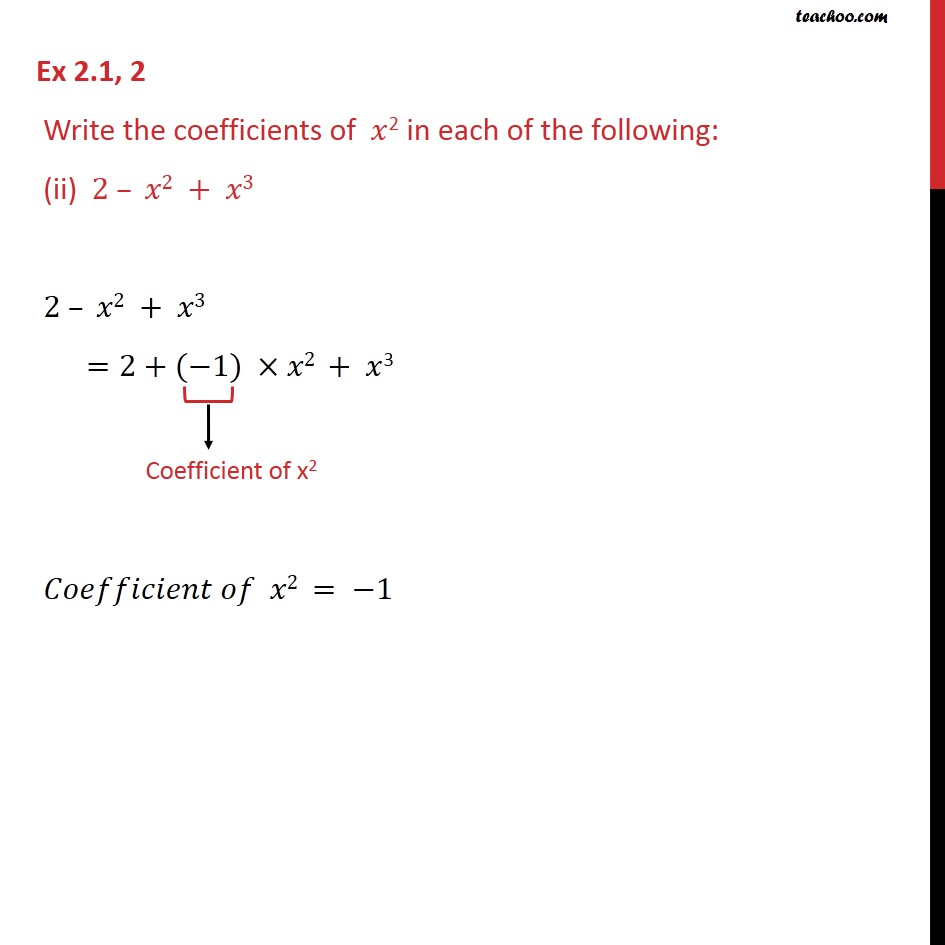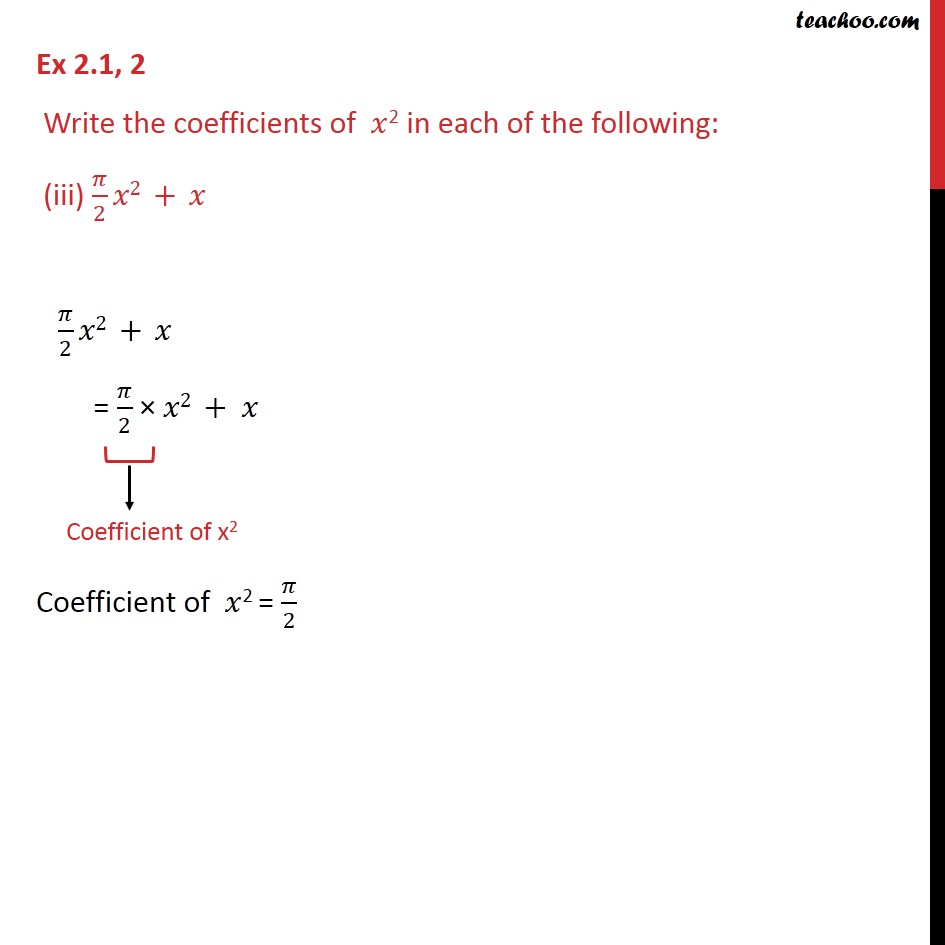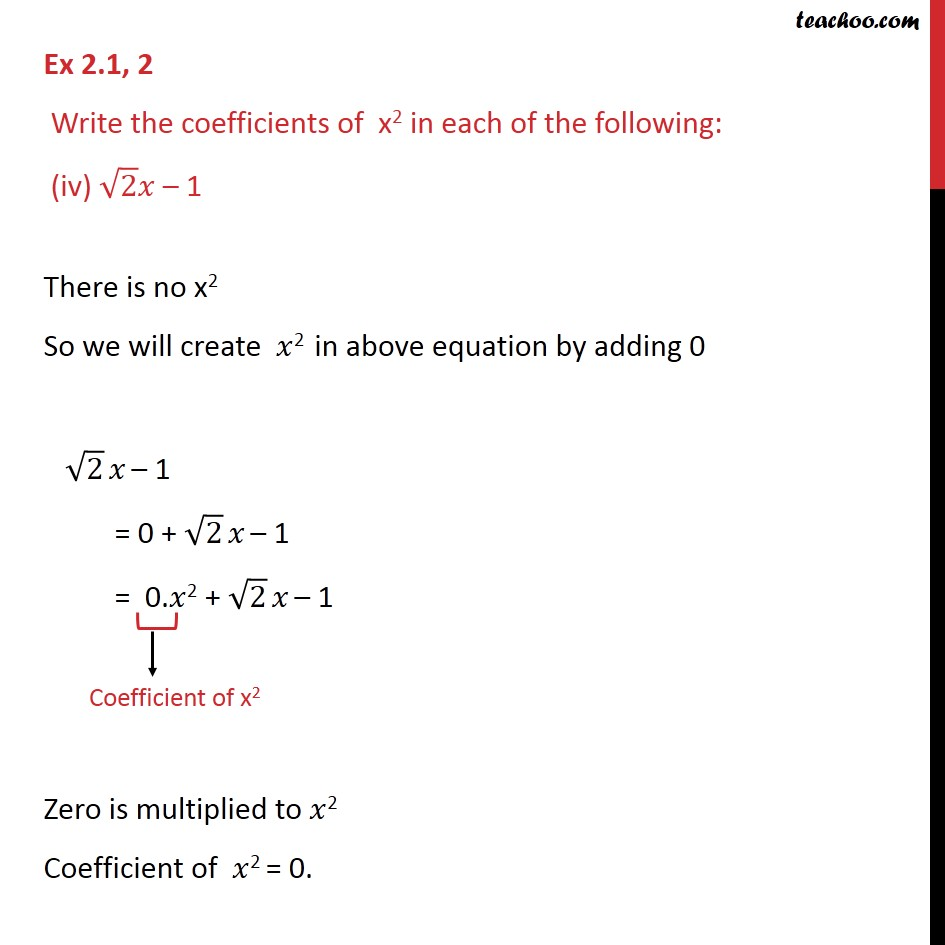Learn all Concepts of Polynomials Class 9 (with VIDEOS). Check - Polynomials Class 91. Chapter 2 Class 9 Polynomials
2. Serial order wise
3. Ex 2.1

Transcript

Ex 2.1, 2 Write the coefficients of 𝑥2 in each of the following: (i) 2+ 𝑥2 + 𝑥 2+ 𝑥2 + 𝑥 = 2+1×𝑥2 + 𝑥 𝐶𝑜𝑒𝑓𝑓𝑖𝑐𝑖𝑒𝑛𝑡 𝑜𝑓 𝑥2 =1 Ex 2.1, 2 Write the coefficients of 𝑥2 in each of the following: (ii) 2 – 𝑥2 + 𝑥3 2 – 𝑥2 + 𝑥3 =2+(−1) ×𝑥2 + 𝑥3 𝐶𝑜𝑒𝑓𝑓𝑖𝑐𝑖𝑒𝑛𝑡 𝑜𝑓 𝑥2 = −1 Ex 2.1, 2 Write the coefficients of 𝑥2 in each of the following: (iii) 𝜋/2 𝑥2 + 𝑥 𝜋/2 𝑥2 + 𝑥 = 𝜋/2 × 𝑥2 + 𝑥 Coefficient of 𝑥2 = 𝜋/2 Ex 2.1, 2 Write the coefficients of x2 in each of the following: (iv) √2 𝑥 – 1 There is no x2 So we will create 𝑥2 in above equation by adding 0 √2 𝑥 – 1 = 0 + √2 𝑥 – 1 = 0.𝑥2 + √2 𝑥 – 1 Zero is multiplied to 𝑥2 Coefficient of 𝑥2 = 0.

Ex 2.1

Chapter 2 Class 9 Polynomials
Serial order wise